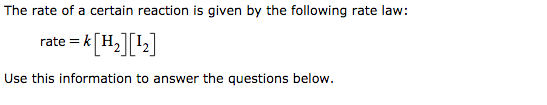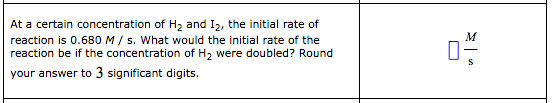# The rate of a certain reaction is given by the following rate law: rate = k[H2][I2] Use this information to answer the questions below. At a certain concentration of H2 and I2, the initial rate of reaction is 0.680 M / s. What would the initial rate of the reaction be if the concentration of H2 were doubled? round your answer to 3 significant digits.